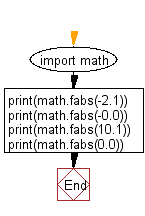﻿ Python Math: Calculate the absolute value of a floating point number - w3resource# Python Math: Calculate the absolute value of a floating point number

## Python Math: Exercise-56 with Solution

Write a Python program to calculate the absolute value of a floating point number.

Sample Solution:-

Python Code:

``````import math

print(math.fabs(-2.1))
print(math.fabs(-0.0))
print(math.fabs(10.1))
print(math.fabs(0.0))
```
```

Sample Output:

```2.1
0.0
10.1
0.0
```

Flowchart:## Visualize Python code execution:

The following tool visualize what the computer is doing step-by-step as it executes the said program:

Python Code Editor:

Have another way to solve this solution? Contribute your code (and comments) through Disqus.

What is the difficulty level of this exercise?

Test your Programming skills with w3resource's quiz.

﻿

```>>> students = [{'name': 'John', 'score': 98}, {'name': 'Mike', 'score': 94}, {'name': 'Jennifer', 'score': 99}]